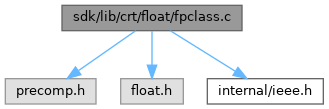ReactOS  0.4.15-dev-2355-gaf9df93
fpclass.c File Reference
`#include <precomp.h>`
`#include <float.h>`
`#include <internal/ieee.h>`
Include dependency graph for fpclass.c:Go to the source code of this file.

## Functions

int _fpclass (double __d)

## ◆ _fpclass()

 int _fpclass ( double __d )

Definition at line 17 of file fpclass.c.

18 {
19  union
20  {
21  double* __d;
22  double_s* d;
23  } d;
24  d.__d = &__d;
25
26
27  /* With 0x7ff, it can only be infinity or NaN */
28  if (d.d->exponent == 0x7ff)
29  {
30  if (d.d->mantissah == 0 && d.d->mantissal == 0)
31  {
32  return (d.d->sign == 0) ? _FPCLASS_PINF : _FPCLASS_NINF;
33  }
34  /* Windows will never return Signaling NaN */
35  else
36  {
37  return _FPCLASS_QNAN;
38  }
39  }
40
41  /* With 0, it can only be zero or denormalized number */
42  if (d.d->exponent == 0)
43  {
44  if (d.d->mantissah == 0 && d.d->mantissal == 0)
45  {
46  return (d.d->sign == 0) ? _FPCLASS_PZ : _FPCLASS_NZ;
47  }
48  else
49  {
50  return (d.d->sign == 0) ? _FPCLASS_PD : _FPCLASS_ND;
51  }
52  }
53  /* Only remain normalized numbers */
54  else
55  {
56  return (d.d->sign == 0) ? _FPCLASS_PN : _FPCLASS_NN;
57  }
58 }
#define _FPCLASS_PN
Definition: float.h:76
#define _FPCLASS_NZ
Definition: float.h:73
#define _FPCLASS_PINF
Definition: float.h:77
#define _FPCLASS_NN
Definition: float.h:71
Definition: ieee.h:10
#define _FPCLASS_PZ
Definition: float.h:74
#define _FPCLASS_PD
Definition: float.h:75
#define _FPCLASS_ND
Definition: float.h:72
#define _FPCLASS_NINF
Definition: float.h:70
#define _FPCLASS_QNAN
Definition: float.h:69
#define d
Definition: ke_i.h:81

Referenced by _yn().